## The Modern Periodic Table

In 1913, Henry Moseley showed that the atomic number of an element is a more fundamental property than its atomic mass. Accordingly, Mendeleev’s Periodic Law was modified and the atomic number was adopted as the basis of the Modern Periodic Table. Modern Periodic Law: ‘Properties of elements are a periodic function of their atomic number.

Elements, when arranged in order of increasing atomic number, lead us to the classification known as the Modern Periodic Table. Prediction of properties of elements could be made with more precision when elements were arranged on the basis of increasing atomic numbers.

Note: See the table on the next page.

## Features of Modern Periodic Table

The Modern Periodic Table has 18 verticaL columns known as ‘groups’ and 7 horizontal rows known as ‘periods’.
Position of Elements in the Modern Periodic Table

In order to find the position of an element in the modern periodic table, the group number and period number of the element is to be found out from its electronic configuration.

## Period

1. The period number of an element is equal to the number of electron shelLs in its atom. If an atom has two occupied shells, it will belong to the second period.

2. The number of elements in a period is fixed by the maximum number of electrons which can be accommodated in the different shells of an atom.

3. The maximum number of electrons that can be accommodated in a shell is given by the formula 2n2, where n is the shell number.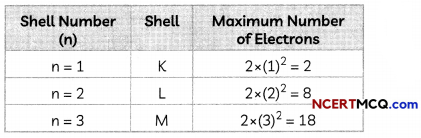4. Each period marks a new electronic shell getting filled.

5. If two or more elements have the same number of valence sheLLs, then they belong to the same period of the periodic table.

• 1st period contains 2 elements and is called very short period.
• 2nd period contains 8 elements and is called short period.
• 3rd period contains 8 elements and is called short period.
• 4th period contains 18 elements and is called long period.
• 5th period contains 18 elements and is also called the long period.
• 6th period contains 32 elements and is called very long period.
• 7th period contains rest of the elements and is incomplete.## Groups

1. The group number of an element having upto two valence electrons is equaL to the number of valence electrons.

2. The group number of an element having more than 2 valence electrons is equal to the number of valence electrons + 10.

• Elements having 1 valence electron are placed in Group 1.
• Elements having 2 valence electrons are placed in Group 2.
• Elements having 3 valence electrons are placed in Group 13.
• ELements having 4 valence electrons are placed in Group 14.
• ELements having 5 valence electrons are placed in Group 15.
• Elements having 6 valence electrons are placed in Group 16.
• Elements having 7 valence electrons are placed in Group 17.
• Elements having 8 valence electrons are placed in Group 18.

3. The elements in a group do not have consecutive atomic numbers.

4. All the elements in a group have similar electronic configurations and show similar properties. ALL elements contain the same number of valence electrons. Groups in the Periodic Table signify an identical outer shell electronic configuration.

5. The number of shells increases as we go down the group.

6. If two or more elements have the same number of valence electrons, then they belong to the same group of the periodic table.Example 1.
Case-Based:
A student studied the Modem Periodic Table and tried to find out the names of elements in Group I, their electronic configuration and similarity in their configuration.
If you look at the long form of the Periodic Table, you will find that the elements Li, Be, B, C, N, O, F, and Ne are present in the second period.
(A) Which of the following statements are incorrect?
(I) The first three elements of Group 1 are H, Li and Na.
(II) Except H, all other elements of Group 1 have one valence electron.
(III) The number of shells remains same on going down the group.
(IV) Groups in the Periodic Table signify an identical outershell electronic configuration.
(a) Both (I) and (III)
(b) Both (II) and (III)
(c) Both (I) and (IV)
(d) Both (II) and (IV)
(b) Both (II) and (III)

Explanation: Hydrogen has one valence electron, just like other elements of Group 1, As we go down a group, a new shell is added in the atom.

For example, consider the elements Li, Na and K having atomic number 3,11 and 19 respectively. They belong to group 1 of the Modern periodic table as they have one electron in their valence shell.

(B) The number of valence electrons of fluorine and Group number to which it belongs is:
(a) Number of valence electrons = 7, Group Number = 1
(b) Number of valence electrons = 9, Group Number = 7
(c) Number of valence electrons = 1, Group Number = 17
(d) Number of valence electrons = 7, Group Number = 17
(d) Number of valence electrons = 7, Group Number = 17

Explanation: The atomic number of fluorine is 9 and its electronic configuration is (2, 7).
As it has two occupied shells, it is placed in second period and as it has seven valence electrons, it is placed in Group 17 of the Modern periodic table.

(C) Why is the element Be placed in the second period and Al in the third period?
The atomic number of Be is 4 and that of Al is 13.
The electronic configuration of be is (2, 2) and that of Al is (2, 8, 3).

As Be has two occupied shells and Al has three occupied shells, Be is placed in second period and Al is pLaced in the third period.

(D) What will be the formula of fluoride of an element E belonging to Group 13 of the Modern Periodic Table?
Fluorine has a valency of 1 as it is a halogen and has seven valence electrons. An element E belonging to group 13 has 3 valence electrons and therefore a valency of 3. Therefore, the formula of the compound formed will be EF3.

(E) Assertion (A): All elements in a period have similar properties.
Reason (R): All elements in a group have identical outer shell electronic configuration.
(a) Both (A) and (R) are true and (R) is the correct explanation of the assertion.
(b) Both (A) and (R) are true, but (R) is not the correct explanation of the assertion.
(c) (A) is true, but (R) is false.
(d) (A) is false, but (R) is true.
(d) (A) is false, but (R) is true.

Explanation: Properties of elements are a periodic function of their atomic number as the properties of elements depend upon their electronic configuration. The elements of a period have the same number of occupied shells but different electronic configuration and hence different properties.

However, elements belonging to the same group have identical outer shell electronic configuration and hence they have similar properties.Example 2.
Case Based:
A student studied the Modem Periodic Table and tried to find out the names of elements in Group I, their electronic configuration and similarity in their configuration.
If you look at the long form of the Periodic Table, you will find that the elements Li, Be, B, C, N, O, F, and Ne are present in the second period.
(A) Which of the following statements are incorrect?
(I) The first three elements of Group 1 are H, Li and Na.
(II) Except H, all other elements of Group 1 have one valence electron.
(III) The number of shells remains same on going down the group.
(IV) Groups in the Periodic Table signify an identical outershell electronic configuration.
(a) Both (I) and (III)
(b) Both (II) and (III)
(c) Both (I) and (IV)
(d) Both (II) and (IV)
(b) Both (II) and (III)

Explanation: Hydrogen has one valence
electron, just like other elements of Group 1, As we go down a group, a new shell is added in the atom.

For example, consider the elements Li, Na and K having atomic number 3,11 and 19 respectively. They belong to group 1 of the Modern periodic table as they have one electron in their valence shell.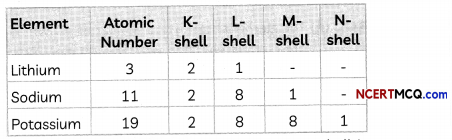As we can see from the above, a new shell is added as we go down a group.

(B) The number of valence electrons of fluorine and Group number to which it belongs is:
(a) Number of valence electrons = 7, Group Number = 1
(b) Number of valence electrons = 9, Group Number = 7
(c) Number of valence electrons = 1, Group Number = 17
(d) Number of valence electrons = 7, Group Number = 17
(d) Number of valence electrons = 7, Group Number = 17

Explanation: The atomic number of fluorine is 9 and its electronic configuration is (2, 7).
As it has two occupied shells, it is placed in second period and as it has seven valence electrons, it is placed in Group 17 of the Modern periodic table.

(C) Why is the element Be placed in the second period and Al in the third period?
The atomic number of Be is 4 and that ofAl is 13.

The electronic configuration of be is (2, 2) and that of Al is (2, 8, 3).
As Be has two occupied sheLls and Al has three occupied shells, Be is placed in second period and Al is placed in the third period.

(D) What will be the formula of fluoride of an element E belonging to Group 13 of the Modern Periodic Table?
Fluorine has a valency of 1 as it is a halogen and has seven valence electrons. An element E belonging to group 13 has 3 valence electrons and therefore a valency of 3. Therefore, the formula of the compound formed will be EF3

(E) Assertion (A): All elements in a period have similar properties.
Reason (R): All elements in a group have identical outer shell electronic configuration.
(a) Both (A) and (R) are true and (R) is the correct explanation of the assertion.
(b) Both (A) and (R) are true, but (R) is not the correct explanation of the assertion.
(c) (A) is true, but (R) is false.
(d) (A) is false, but (R) is true.
(d) (A) is false, but (R) is true.

Explanation: Properties of elements are a periodic function of their atomic number as the properties of elements depend upon their electronic configuration. The elements of a period have the same number of occupied shells but different electronic configuration and hence different properties.

However, elements belonging to the same group have identical outer shell electronic configuration and hence they have similar properties.Example 3.
Which element has:
(A) two shells, both of which are completely filled with electrons?
The element having two shells, both of which are completely filled will have an electronic configuration of (2,8). Therefore, the element Neon (Atomic number = 10) has two shells, both of which are completely filled.

(B) the electronic configuration 2, 8, 2?
Electronic configuration (2, 8, 2) means that the atomic number of the element is 12, which is that of Magnesium (Mg).

(C) a total of three shells, with four electrons in its valence shell?
Element having three shells, with four electrons in its valence shell or M shell will have the electronic configuration 2, 8, 4. Therefore, the atomic number of the element is 14 and the element is silicon which belongs to group 14.

(D) a total of two shells, with three electrons in its valence shell?
Element having two shells, with three electrons in its valence shell or L shell will have the electronic configuration 2, 3. Therefore, the atomic number of the element is 5 and the element is boron which belongs to group 13.

(E) twice as many electrons in its second shell as in its first shell?
Element having twice as many electrons in its second shell (L shell) as in its first shell (K shell) will have the electronic configuration 2, 4. Therefore, the atomic number of the element is 6 and the element is carbon which belongs to group 14.

Arrangement of elements in the modern periodic table on the basis of the electronic configurations of their atoms Number of valence electronsPosition of Hydrogen: There is an anomaly when it comes to the position of hydrogen because it can be placed either in group 1 or group 17 in the first period.
Hydrogen has been placed at the top of Group I, above the alkali metals because the electronic configuration of hydrogen is similar to those of alkali metals. Both have 1 valence electron each. Since the size of hydrogen atom is much smaller than that of aLkali metals, many properties of hydrogen are different from those of alkali metals.## Explanation of the Anomalies of Mendeleev’s Classification of Elements

1. Explanation of the position of hydrogen: A unique position has been given to hydrogen. It has been placed at the top left corner in group 1, period 1 because of its unique characteristics.

2. Explanation for the position of isotopes: Since all the isotopes of an element have the same atomic number, they are put at one place in the same group of the periodic table.

3. Explanation for the Position of Cobalt and Nickel: Since the elements are arranged according to their atomic number, and the atomic number of cobalt is 27 while that of nickel is 28, so cobalt with lower atomic number should come first and nickel with higher atomic number should come later.

Comparison of Mendeleev’s Periodic table and Modern periodic table

 Mendeleev’s Periodic Table Modern Periodic Table 1. Mendeleev’s periodic table is based on atomic mass. 1. Modern periodic table is based on atomic number. 2. There were gaps for undiscovered elements. 2. Modern periodic table maintains uniformity. 3. Noble gases were not placed (as they are not discovered at that time) 3. Noble gases in a separate group named as group-18. 4. There are a total of 8 groups and 6 periods 4. There are a total of 18 groups and 7 periods

Example 4.
How could the Modem Periodic Table remove various anomalies of Mendeleev’s Periodic Table? [NCERT]
The Modern Periodic Table is based on grouping elements with similar properties on the basis of their atomic numbers, whereas Mendeleev’s periodic table was based on atomic masses. This led to certain anomalies, such as the position of hydrogen, position of isotopes and the fact that atomic masses do not increase in a regular manner on moving from one element to the next.

1. Hydrogen has been allotted a unique position, i.e., it is placed at the top of alkali metals in the first group based on similarity in electronic configuration with alkali metals.
2. The anomaly regarding position of isotopes of an element have been resolved as isotopes of an element have the same atomic number and so they have been allotted the same position in the modern periodic table.
3. The position of Cobalt and nickel is now justified as the atomic number of Cobalt is less than an atomic number of nickeland hence it is placed at 9th group whereas Nickel is placed at the 10th group.
4. As the elements have been arranged in the order of increasing atomic number, the number of elements Lying between any two elements can easily be predicted.

## Trends in the Modern Periodic Table Valency

The valency of an element is its combining capacity with other atoms in order to attain the nearest inert gas configuration. It is related to the number of valence electrons present in the atom of an element.

Variation along a period: On moving from left to right, the valency increases from 1 to 4 and then decreases to 0 from group 15 to 18 as the valency is determined by the number of valence electrons.

Example: Valency of Na (Z = 11) is 1 as its electronic configuration is 2, 8, 1 and it attains its nearest inert gas configuration by losing one electron.

Valency of O (Z = 8) is 2, as its electronic configuration is (2, 6) and it requires two electrons to attain its nearest inert gas configuration.

Variation along a group: All elements in a group have the same valency as elements in the same group have the same number of valence electrons.

• Valency of group 1 elements = Number of valence electrons = 1
• Valency of group 2 elements = Number of valence electrons = 2
• Valency of group 13 elements = Number of vaLence electrons = 3
• Valency of group 14 elements = Number of valence electrons = 4
• Valency of group 15 elements = 8 – Number of valence electrons = 3
• Valency of group 16 elements = 8 – Number of valence electrons = 2
• Valency of group 17 elements = 8 – Number of valence electrons = 1
• Valency of group 18 eLements = 8 – Number of valence electrons = 0

Example: Elements Li, Na and K belong to group 1 as they all have one valence electron and hence have valency 1.
Li: 2,1
Na: 2,8,1
K: 2, 8, 8,1

Elements F, Cl and Br belong to group 17 as they all have seven valence electrons and hence have valency 1 (8 – 7).
F: 2,7
CL: 2, 8, 7
Br: 2, 8, 8,7Example 5.
Element X forms a chloride with the formula XCl2, which is a solid with a high melting point. X would most likely be in the same group of the Periodic Table as
(a) Na
(b) Mg
(c) Al
(d) Si
(b) Mg

Explanation: As the formula of chloride of element X is XCI2, and valency of Cl is 1, it means that valency of X is 2. Since metals combine with halogens to form metal halides, X is a metaLwith valency 2 and hence occupies group 2 of the periodic table.

Out of the elements given Na belongs to group 1, Mg to group 2, Al to Group 13 and Si to group 14 of the periodic table.

Example 6.
In the Modem Periodic Table, calcium (atomic number 20) is surrounded by elements with atomic numbers 12, 19, 21 and 38. Which of these have physical and chemical properties resembling calcium?
To find the elements having similar physical and chemical properties as calcium, we have to find their electronic configuration.

We observe that the elements Mg and Sr also have two valence electrons just like Ca. Therefore, Calcium will have properties similar to both Mg and Sr,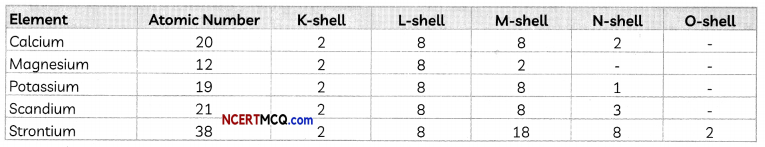## Atomic size

The size of an atom refers to the radius of atom and is the distance between the centre of the nucleus and the outermost shell of an isolated atom. It is expressed in picometer.
1 picometer = 10-12 m

Variation along a period: On moving from left to right in a period, the size of atoms decreases.

Reason: When we move from left to right in a period, the number of electrons and protons increases. Due to the large positive charge on the nucleus, electrons are pulled more strongly towards the nucleus.

Variation along a group: The size of an atom (radius) increases as we go down in a group Reason: When going from top to bottom in a group, a new shell is added to the atoms which increases the distance between the valence electrons and the nucleus. So, the effective nuclear charge experienced by the valence electrons decreases.## Metallic and Non-metallic Character

Metals are the elements that have 1, 2 or 3 electrons in their valence shell and lose electrons easily to form positive ions or cations. They are present on the Left side and centre of the periodic table. They are calLed electropositive elements as they have a tendency of losing electrons.

Non-Metals are the elements that have 4, 5, 6 or 7 electrons in their valence shell and gain electrons to form negative ions or anions. They are present on the right side of the periodic table. They are called electronegative elements as they have a tendency of gaining electrons.

Metalloids: There are some elements known as metalloids that exhibit properties of both metals and non-metals.
In the Modern Periodic Table, a zig-zag line separates metals from non-metals. The borderline elements – boron, silicon, germanium, arsenic, antimony, tellurium and polonium – are intermediate in properties and are called metalloids or semi-metals.

Variation along a period: The metallic character decreases and non-metallic character increases as we move from left to right in a period.

Reason: When we move from Left to right in a period, the electropositive character of elements decreases, but the electronegative character increases. Due to the large positive charge on the nucleus, electrons are pulled more strongly towards the nucleus.

Example:
Consider the elements of 3rd period. The variation of metalLic character is shown below: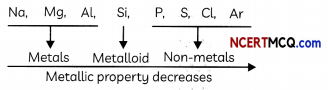Variation along with a group: The metallic character increases and non-metallic character decreases as we move from top to bottom in a group.
Reason: When we go down a group, the electropositive character of elements increases as the tendency of an atom to tose electrons increases as the effective nuclear charge experienced by the valence electrons decrease. The electronegative character decreases down o group as the tendency to gain electrons decreases.Example 7.
Case Based:
Let us study how the tendency ro lose electrons Will change in a group and how this tendency will change
in a period.

In the periodic table, study the change in the tendency to gain electrons as we go from left to right across a period and as we go down a group.

(A) Group 1 eLements when, arranged in increasing order of their reactivity is:
(a) Na < Li < K < Rb < Cs
(b) Cs Rb < K < Na < Li
(c) Li < Na < K < Rb <Cs
(d) K < Na < Li < Rb <Cs
(c) Li < Na < K < Rb < Cs

Explanation: The reactivity of a metal depends upon its electropositivity, i.e., its ability to form cations by losing electrons. As we move down a group, a new shell is added in the atom and the outermost electrons are farther away from the nucleus due to which the effective nuclear charge experienced by valence electrons decreases. Therefore, an atom can easily lose electrons. Hence metallic character increases down a group. As Li is at the top of Group 1 and Cs is at the bottom of the group, Cs is more reactive than Li and reactivity increases from top to bottom in a group.

(B) The variation of electronegativity values for the first 20 elements is shown below: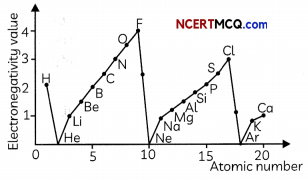Study the graph above and select the correct statement:
(I) The alkali metals are the most electronegative elements in a period.
(II) The electronegativity increases as we go down a group
(III) The electronegativity increases as we move from left to right along a period.
(IV) In a given period, the halogens are the most electronegative elements.
(a) Both (I) and (III)
(b) Both (I) and (IV)
(c) Both (II) and (IV)
(d) Both (III) and (IV)
(d) Both (III) and (IV)

Explanation: As we move from left to right along a period, the eLectro negativity increases as the ability of atoms to gain electrons increases due to decrease in atomic size. This is due to the increase in effective nuclear charge as electrons are added in the same shell in a period. Since halogens are in group 17, they are the most electronegative elements in a period and alkali eLements are the most electro-positive elements in a period. The electronegativity decreases as we move from top to bottom in a group which is due to the decrease in the effective nuclear charge experienced by valence electrons due to increase in atomic size.

(C) What happens to tendency to gain electron down the group and why?
The tendency to gain electron decreases down a group because as the atomic size increases, the effective nuclear charge experienced by valence electrons decreases as the outermost electrons are farther away from the nucleus.

(D) Arrange the elements F, Cl, Br and I in increasing order of reactivity.
I < Br < Cl <F.
Explanation: The elements F, Cl, Br and I belong to Group 17 of the modern periodic table.
The electronic configuration of F, Cl, Br and I is given below:We observe that a new shell is added when we move down the group due to which the effective nuclear charge experienced by the valence electrons decreases and hence reactivity decreases.

(E) Assertion (A): The tendency to lose electrons decreases as we go from left to right in a period.
Reason (R): The effective nuclear charge acting on the valence shell electrons increases across a period.
(a) Both (A) and (R) are true and (R) is the correct explanation of the assertion.
(b) Both (A) and (R) are true, but (R) is not the correct explanation of the assertion.
(c) (A) is true, but (R) is false.
(d) (A) is false, but (R) is true.
(a) Both (A) and (R) are true and (R) is the correct explanation of the (A).Example 8.
By considering their position in the Periodic Table, which one of the following elements would you expect to have maximum metallic characteristic? Ga Ge As Se Be
The element Be is placed in the 2nd period and 2nd group of the periodic table. Whereas the elements Ga, Ge, As and Se are placed in 4th period, group 13 to 16 respectively.

As the metals are placed on the left side of the periodic table and non-metals on the right side, we can say that Be is the most metallic of these elements.

Example 9.
Nitrogen (atomic number 7) and phosphorus (atomic number 15) belong to group 15 of the Periodic Table. Write the electronic configuration of these two elements. Which of these will be more electronegative? Why?
The electronic configuration of the elements is given below: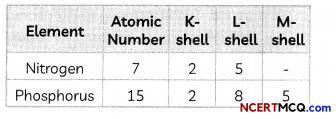Electronegativity is the ability to gain electrons. Nitrogen will be more electronegative than phosphorus as nitrogen has smaller atomic size than phosphorus as it has 2 shells whereas phosphorus has three shells. As the number of shells increases, the distance between the nucleus and valence shell increases due to which it becomes more difficult for an atom to gain electrons.

## Chemical Reactivity

The chemical reactivity of an element depends upon the electronic configuration of the atom of the alement.
Variation along a period: The chemical reactivity of elements first decreases and then increases when we move from left to right in a period.

Variation along a group: The chemical reactivity of metals increases on going down in a group.
The chemical reactivity of non-metals decreases on going down in a group.

Example 10.
(A) Lithium, sodium, potassium are all metals that react with water to liberate hydrogen gas. Is there any similarity in the atoms of these elements?
Yes, Li, Na and K belong to group 1 of the modern periodic table as atoms of all these metals have one valence electron in their outermost shell.
Electronic configuration of Li (Atomic Number=3) is 2,1
Electronic configuration of Na (Atomic Number = 11) is 2, 8,1
Electronic configuration of K (Atomic Number = 19) is 2, 8, 8,1

(B) Helium is an unreactive gas and neon is a gas of extremely low reactivity. What, if anything, do their atoms have in common?
Both He and Ne are inert gases and have a completely filled valence shell which expLains their chemical inertness.
Electronic configuration of He (Atomic Number = 2): 2
Electronic configuration of Ne (Atomic Number = 10): 2, 8

## Nature of Oxides

Variation along a period: The basic nature of oxides decreases and the acidic nature increases as we move from left to right as metal oxides are basic in nature and oxides of non-metals are acidic in nature. Variation along a group: There is no change in the nature of oxides as we go down in a group as aLl elements of the same group have similar chemical nature.Example 11.
Which of the following statements is not a correct statement about the trends when going from left to right across the periods of Periodic Table.
(a) The elements become less metallic in nature.
(b) The number of valence electrons increases.
(c) The atoms lose their electrons more easily.
(d) The oxides become more acidic.
(c) The atoms lose their electrons more easily. Explanation: On moving from left to right along a period, the metallic character decreases. This is because when we move from left to right in a period, the tendency to lose electrons decreases as the effective nuclear charge on the valence electrons increases.

However, the number of valence electrons increases and the non-metallic character also increases because of the increase in the electronegativity. Further, oxides of non-metals are acidic whereas metal oxides are basic in nature.

Example 12.
The position of three elements A, B and C in the Periodic Table are shown below: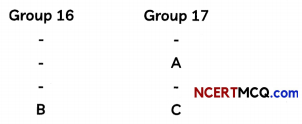(A) State whether A is a metal or non -metal.
Since A belongs to group 17 and the number of valence electrons is 7, it has a valency of 1 it will gain one electron to complete its octet. Therefore, A is a non-metal.

(B) State whether C is more reactive or less reactive than A.
C is less reactive than A because as we move down in a group, the reactivity of non-metals decreases. This is because, as we move in a group, the atomic size increases as a new shell is added due to which the distance between the nucleus and valence electrons increases, and hence electronegativity decreases.

(C) Will C be larger or smaller in size than B?
C is smaller in size than B because B and C both are placed in the same period and the size decreases as one moves from left to right in a period due to an increase in effective nuclear charge.

(D) Which type of ion, cation or anion, will be formed by element A?
A will form anion because it is a non-metal and will gain one electron to complete its octet.

## Merits of the Modern Periodic Table

1. It is based on the atomic number of elements which is the most fundamental property of elements.
2. It helps us understand why elements in a group show similar properties but elements in different groups show different properties.
3. It explains the reasons for the periodicity in properties of elements.
4. The modern periodic table tells us why the properties of elements are repeated after 2, 8,18, and 32 elements.
5. There are no anomalies in the arrangement of elements in the modern periodic table.

## Advantages of the Periodic Table:

1. The periodic table has made the study of chemistry systematic and easy.
2. It is easier to remember the properties of an element if its position in the periodic table is known.
3. The type of compounds formed by an element can be predicted by knowing its position in the periodic table.
4. A periodic table chart is used as a teaching aid in chemistry in schools and colleges.